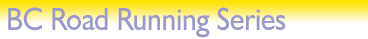# Rankings

## Predict Your Score Calculator

This calculator lets you input a race time to see what score it would get you.
If you want to do the opposite (see what time you need to run for a certain score), click here.

 Race length in km (you can use a decimal point): km My time (hrs:min:sec): : : Gender: Male Female My score (out of 1000):

The formula is:

S = (141000/T) x D1.0631 for males; S = (167000/T) x D1.0425 for females

where T is the time in seconds, D is the race distance in km, and S is the calculated score.

Top